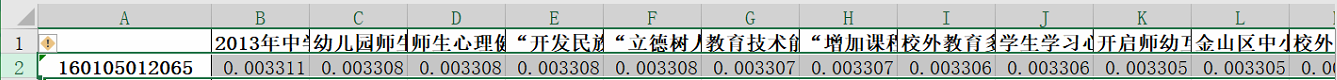# 理论介绍

## 协同过滤算法简介

1. 找到与目标兴趣相似的用户集群
2. 找到这个集合中用户喜欢的、并且目标用户没有听说过的商品推荐给目标用户。## 常用的相似度计算方法

#### 欧式距离（Euclidean Distance）

d = ( x 1 − x 2 ) 2 + ( y 1 − y 2 ) 2 d=\sqrt{(x_{1}-x_{2})^{2}+(y_{1}-y_{2})^{2}}

d = ( x 1 − x 2 ) 2 + ( y 1 − y 2 ) 2 + ( z 1 − z 2 ) 2 d=\sqrt{(x_{1}-x_{2})^{2}+(y_{1}-y_{2})^{2}+(z_{1}-z_{2})^{2}}

d x y = ∑ i = 1 n ( x i − y i ) 2 d_{xy}=\sqrt{\sum_{i=1}^{n} (x_{i}-y_{i})^{2}}

s i m ( x , y ) = 1 1 + d ( x , y ) sim(x,y)=\frac{1}{1+d(x,y)}

#### 余弦相似度（Cosine）Cosine相似度是根据两个向量的夹角计算相似度，夹角越小相似度越高。

cos ⁡ ( θ ) = ∑ k = 1 n x 1 k x 2 k ∑ k = 1 n x 1 k 2 ∑ k = 1 n x 2 k 2 \cos (\theta)=\frac{\sum_{k=1}^{n} x_{1 k} x_{2 k}}{\sqrt{\sum_{k=1}^{n} x_{1 k}^{2}} \sqrt{\sum_{k=1}^{n} x_{2 k}^{2}}}

sim ⁡ x , Y = X Y ∥ X ∥ ⋅ ∥ Y ∥ = ∑ i = 1 n ( x i ∗ y i ) ∑ i = 1 n ( x i ) 2 ⋅ Σ i = 1 n ( y i ) 2 \operatorname{sim}_{x, Y}=\frac{X Y}{\|X\| \cdot\|Y\|}=\frac{\sum_{i=1}^{n}\left(x_{i} * y_{i}\right)}{\sqrt{\sum_{i=1}^{n}\left(x_{i}\right)^{2}} \cdot \sqrt{\Sigma_{i=1}^{n}\left(y_{i}\right)^{2}}}

sim ⁡ x , y ≠ sim ⁡ y , x \operatorname{sim}_{x, y} \neq \operatorname{sim}_{y, x}

# 实现过程

### UserCF和ItemCF的比较

Item CF是利用课程间的相似性来推荐的，所以假如教师的数量远远超过课程的数量，那么可以考虑使用Item CF，比如购物网站，因其课程的数据相对稳定，因此计算课程的相似度时不但计算量较小，而且不必频繁更新;User CF更适合做新闻、博客或者微内容的推荐系统，因为其内容更新频率非常高，特别是在社交网络中，User CF是一个更好的选择，可以增加教师对推荐解释的信服程度。

### 基于教师(user-based)的协同过滤实现过程

1. 计算就是将一个教师对所有课程的偏好作为一个向量来计算教师之间的相似度（找领居）
2. 找到 K 邻居后，根据邻居的相似度权重以及他们对课程的偏好，（看邻居偏好）
3. 预测当前教师没有偏好的未涉及课程，计算得到一个排序的课程列表作为推荐。（召回排序）### 基于课程(item-based)的协同过滤实现过程

1. 从计算的角度看，就是将所有教师对某个课程的偏好作为一个向量来计算课程之间的相似度，（找教师偏好的“共性”课程）
2. 得到课程的相似课程后，根据教师历史的偏好预测当前教师还没有表示偏好的课程，计算得到一个排序的课程列表作为推荐。（预测召回，排序）# 案例实战import numpy as np
import pandas as pd

# 读取原始数据文件

header = ['user_id', 'item_id', 'rating', 'timestamp']


# 数据预处理
# 教师对所有课程只有选上1和未选0两种状态，顾选课为1
# 1.进修编号及课程按照从0开始重新编码，为了后续矩阵运算
user_list = pd.DataFrame({'进修编号': df.user_id.unique(), '矩阵编号': np.arange(df.user_id.unique().shape)})
items_list = pd.DataFrame({'课程名称': df.item_id.unique(), '矩阵编号': np.arange(df.item_id.unique().shape)})
user_list.to_excel('../输出数据/进修编号矩阵编码.xlsx', index=False)
items_list.to_excel('../输出数据/课程名称矩阵编码.xlsx', index=False)

i = 0
for line in user_list.itertuples():     #.itertuples()可以使user_list分成多个对象
df.loc[(df['user_id'] == line), 'user_id'] = line
print('重新编码教师，已完成',round(i/user_list.shape*100.00,2), '%', end="\r")
i+=1

i = 0
for line in items_list.itertuples():
df.loc[(df['item_id'] == line), 'item_id'] = line
print('重新编码课程，已完成',round(i/items_list.shape*100.00,2), '%', end="\r")
i+=1

print(df)
df.to_excel('../输出数据/矩阵数据.xlsx', index=False)# 数据计算
# 计算唯一教师和课程数量
n_users = df.user_id.unique().shape
n_items = df.item_id.unique().shape
print('Number of teachers = ' + str(n_users) + ' | Number of courses = ' + str(n_items))# 使用scikit-learn库将数据集分割成测试和训练。train_test_split根据测试样本的比例（test_size）
# ，本例中是0.2，来将数据混洗并分割成两个数据集
from sklearn.model_selection import train_test_split

train_data, test_data = train_test_split(df, test_size=0.2)

# 基于内容的协同过滤
# 第一步是创建uesr-item矩阵，此处需创建训练和测试两个UI矩阵
# 初始化一个user行，items列
train_data_matrix = np.zeros((n_users, n_items))
for line in train_data.itertuples():
train_data_matrix[line, line] = line

# print(train_data_matrix)
# a = pd.DataFrame(train_data_matrix)
# # a.to_excel('../输出数据/test.xlsx', index=False)
# print(a)

test_data_matrix = np.zeros((n_users, n_items))
for line in test_data.itertuples():
test_data_matrix[line, line] = line


# 计算相似度
# 使用sklearn的pairwise_distances函数来计算余弦相似性
from sklearn.metrics.pairwise import pairwise_distances
# 计算用户相似度
user_similarity = pairwise_distances(train_data_matrix, metric='cosine')
# 计算物品相似度
item_similarity = pairwise_distances(train_data_matrix.T, metric='cosine')


# 预测
def predict(ratings, similarity, type='user'):
# 基于用户相似度矩阵的
if type == 'user':
mean_user_rating = ratings.mean(axis=1)
# You use np.newaxis so that mean_user_rating has same format as ratings
ratings_diff = (ratings - mean_user_rating[:, np.newaxis])
pred = mean_user_rating[:, np.newaxis] + similarity.dot(ratings_diff) / np.array(
[np.abs(similarity).sum(axis=1)]).T

# 基于物品相似度矩阵
elif type == 'item':
pred = ratings.dot(similarity) / np.array([np.abs(similarity).sum(axis=1)])
return pred

# 预测结果
item_prediction = predict(train_data_matrix, item_similarity, type='item')
user_prediction = predict(train_data_matrix, user_similarity, type='user')

user = user_list.进修编号.array     #提取教师原始编号
items = items_list.课程名称.array   #提取课程原始编号

item_prediction = pd.DataFrame(item_prediction, index=user, columns=items)
item_prediction_forty = item_prediction[0:40]

user_prediction = pd.DataFrame(user_prediction, index=user, columns=items)
user_prediction_forty = user_prediction[0:40]

user_prediction_forty.to_excel('../输出数据/基于用户预测结果40.xlsx')
item_prediction_forty.to_excel('../输出数据/基于课程预测结果40.xlsx')##### 进修编号：160105012065##### 进修编号：160105004059#### 感谢阅读~

09-29
01-12326006-12
03-306万+
05-221万+
12-30556
10-201321
07-042万+
11-127万+
09-071万+
06-082781
05-033058
09-183360
01-052万+
08-05183
05-20816
01-161万+
03-25333
05-141277
08-171659
07-04446